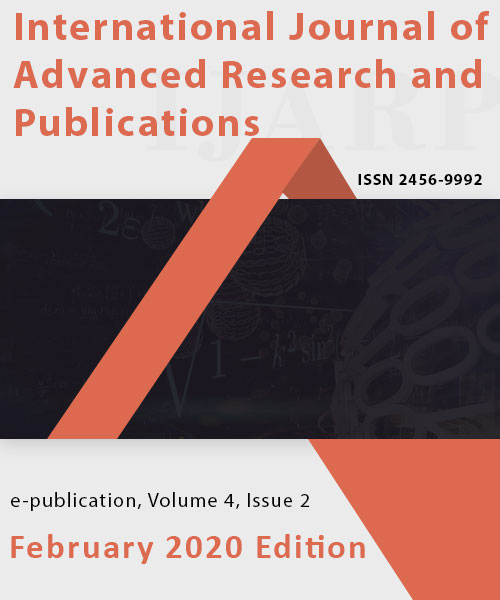# International Journal of Advanced Research and Publications

## High Quality Publications & World Wide Indexing!

#### A Cauchy Problem For A Class Of Nonlinear Hyperbolic First Order Partial Differential Equation In A Banach SpaceVolume 4 - Issue 2, February 2020 Edition

Author(s)
O. Echude, B. Balarabe, H. Mohammed
Keywords
Accretive, Cauchy Problem, Non-linear PDE, Hyperbolic
Abstract
The ultimate work done in this research is the vast and active field of nonlinear hyperbolic first order partial differential equations. A class of nonlinear hyperbolic first order partial differential equation in a Banach space was investigated by converting it to Cauchy-like equation, than show that the operator A is accretive, M-accretive and thus admit a solution.
References
 D. K. Gvazava (originator), Quasi-linear hyperbolic equations and systems. Encyclopedia of Mathematics. URP:http://www.encyclopediaofmath.org/index.php?title=Quasilinear_hyperbolic_equations_and_systems&oldid=16213

 E. Levi, "Sur problema di Cauchy per le equazioni a caratteristiche reali e distinti" Rend. R. Acad. Lincei (5) , 17 (1908) pp. 331â€“339

 M. O. Egwurube, & E. J. D. Garba, â€œA Cauchy problem for a quasi-linear hyperbolic first-order partial differential equationâ€. Journal of Nigeria Association of Mathematical Physics, 7, 29 â€“ 32. 2003.

 A. U. Bawa, M. O. Egwurube, & M. M. Ahmad, â€œA quasi-linear parabolic partial differential equation with accretive propertyâ€. Tanzania Journal of Natural and Applied Sciences. 3, 433-438, 2012.

 O. Echude, M. T. Y. Kadzai and A. A. Abubakar, â€œA non-linear partial differential accretive operator in a closed, bounded and continuous domainâ€. International Journal of Advanced Research and Publications (IJARP); Volume 1, (2017), 84-87

 F. E. Browder, â€œNon-linear mappings of non-expansive and accretive type in Banach spaceâ€. Bulletin of the American Mathematical Society, 73, 875 â€“ 882, 1967.

 F. E. Browder, â€œNonlinear monotone and accretive operators in Banach Spacesâ€. in Proceedings of the Symposium on Nonlinear Functional Analysis (August 1968) (American Mathematical Society). 61, 388-39, 1968.